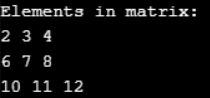# Functions using Array in C

## Functions using Array in C

In this article, I am going to discuss Functions using Array in C with Examples. Please read our previous articles, where we discussed the Multi-Dimensional Array in C Language with examples.

##### How to pass and return an array from a function in C?

Functions make our program modular and maintainable. Big applications can have hundreds of functions. The array is a data structure to store a homogeneous collection of data. Arrays are equally important as functions. In programming, we often use arrays and functions together. Here I will explain how to pass and return an array from a function in C programming.

##### How to pass a single dimensional array to function?

In C you can pass single-dimensional arrays in two ways. You can either pass it directly to a function. Or you can also pass it as a pointer to the array.

###### Passing array directly to function
```#include <stdio.h>
void printArray(int arr[], int size)
{
int i;
printf("Array elements are: ");
for(i = 0; i < size; i++)
{
printf("%d, ", arr[i]);
}
}
int main()
{
int arr;
printArray(arr, 5);    // Pass array directly to function printArray
return 0;
}```

Output: Array elements are: 0, 0, -858062720, 22008, -1127637968,

###### Passing array using a pointer to a function in C

Since array and pointers are closely related to each other. Hence you can also pass an array to function as a pointer.

```#include <stdio.h>
void printArray(int * arr, int size)
{
int i;
printf("Array elements are: ");
for(i = 0; i < size; i++)
{
printf("%d, ", arr[i]);
}
}
int main()
{
int arr;
printArray(arr, 5);    // Pass array directly to function printArray
return 0;
}```

Output: Array elements are: 0, 0, -295874432, 22032, -735842928,

Note: Arrays in C are passed as a reference, not by value. This means any changes to an array within the function will also persist outside the function.

##### How to return a single dimensional array from the function?

In C you cannot return an array directly from a function. But that does not impose a restriction on the C language. There are two ways to return an array indirectly from a function.

###### Return pointer pointing at array from a function

C does not allow you to return an array directly from the function. However, you can return a pointer to the array from the function.

```#include <stdio.h>
/**
* Function to return an array using pointers.
* @return 	Pointer to array
*/
int * getArray()
{
int num[] = {1, 2, 3, 4, 5};
int i;
printf("Array inside function: ");
// Print value of each array element
for (i = 0; i < 5; ++i)
{
printf("%d\n", num[i]);
}
return num;
}
int main()
{
int i;
// Pointer to store array
int * num;
// Call getArray function to get pointer to array
num = getArray();
printf("Array outside function: \n");
// Print value of each array element
for (i = 0; i < 5; ++i)
{
printf("%d\n", num[i]);
}
return 0;
}```

Output: C compiler reports a warning message on the compilation of the above program.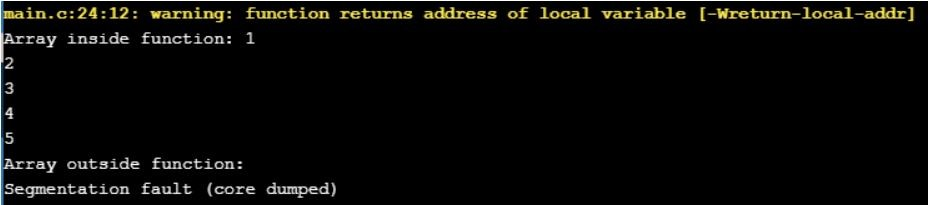It complains about returning the address of a local variable. We can return the value of a local variable but it is illegal to return a memory location that is allocated within the function on the stack. Since, after program control is returned from the function all variables allocated on the stack within the function are freed. Hence, returning a memory location that is already released will point at no man’s land.

##### Pass the returned array as a parameter in C

Arrays in C are passed by reference, hence any changes made to an array passed as an argument persists after the function. So, you can accept the output array you need to return, as a parameter to the function.

```#include <stdio.h>
#define MAX_SIZE 10
/* Function delcaration to initialize array and return */
void getArray(int arr[], int size);
int main()
{
int arr[MAX_SIZE];
int i;
// Call function to initialize array.
getArray(arr, MAX_SIZE);
printf("\n\nArray outside function: \n");
for (i = 0; i < MAX_SIZE; i++)
{
printf("%d ", arr[i]);
}
return 0;
}
/**
* Function to initialize array.
*
* @arr     Integer array to initialize and return.
* @size    Size of the array.
*/
void getArray(int arr[], int size)
{
int i;
printf("Enter elements in array: ");
for (i = 0; i < size; i++)
{
scanf("%d", &arr[i]);
}

printf("\n\nArray inside function: \n");
for (i = 0; i < size; i++)
{
printf("%d ", arr[i]);
}
}```
###### Output: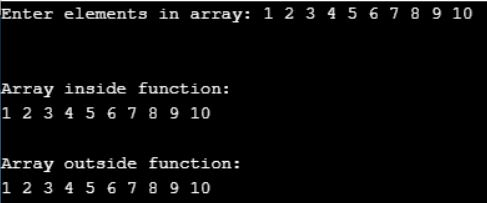##### How to pass a multi-dimensional array to function?

Multi-dimensional arrays are passed in the same fashion as single-dimensional. This means you can pass the multi-dimensional array to a function in two ways.

###### Passing multi-dimensional array directly to function

This is the simplest way to pass a multi-dimensional array to functions. Pass the array as other variables.

```#include <stdio.h>
#define ROWS 3
#define COLS 3
/* Function declaration to print two dimensional array */
void printMatrix(int mat[][COLS]);
int main()
{
int mat[ROWS][COLS] = {
{1, 2, 3},
{4, 5, 6},
{7, 8, 9}
};
// Print elements of matrix using function
printMatrix(mat);
return 0;
}
/**
* Function to accept two-dimensional array and print
* its elements.
* @mat     Two-dimensional integer array to print.
*/
void printMatrix(int mat[][COLS])
{
int i, j;
// Print elements of two-dimensional array.
printf("Elements in matrix: \n");
for (i = 0; i < ROWS; i++)
{
for (j = 0; j < COLS; j++)
{
printf("%d ", mat[i][j]);
}
printf("\n");
}
}```
###### Output: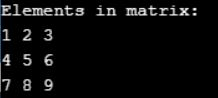###### Passing a multi-dimensional array to function using a pointer
```#include <stdio.h>
#define ROWS 3
#define COLS 3
/* Function declaration */
void inputMatrix(int (*mat)[COLS]);
void printMatrix(int mat[][COLS]);
int main()
{
int mat[ROWS][COLS];
// Input elements in matrix using function
inputMatrix(mat);
// Print elements of matrix using function
printMatrix(mat);
return 0;
}
/**
* Function to accept a two-dimensional array and input
* elements in matrix from user.
*
* @mat     Two-dimensional integer array to store user input.
*/
void inputMatrix(int (*mat)[COLS])
{
int i, j;
// Input elements in 2D matrix
printf("Enter elements in 2D matrix: \n");
for (i = 0; i < ROWS; i++)
{
for (j = 0; j < COLS; j++)
{
scanf("%d", (*(mat + i) + j));
}
}
}
/**
* Function to accept a two-dimensional array and print
* its elements.
*
* @mat     Two-dimensional integer array to print.
*/
void printMatrix(int (*mat)[COLS])
{
int i, j;
// Print elements of two-dimensional array.
printf("Elements in matrix: \n");
for (i = 0; i < ROWS; i++)
{
for (j = 0; j < COLS; j++)
{
printf("%d ", *(*(mat + i) + j));
}
printf("\n");
}
}```
###### Output: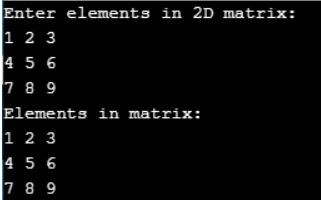Note: int (*mat)[COLS] and int * mat[COLS] both are different. The first is a pointer to an array whereas the second is an array of pointers.

##### How to return a multi-dimensional array from a function?

Returning a multi-dimensional array from the function is similar to returning a single-dimensional array. This means you can either return a pointer to an array or pass the array to return as a function parameter.

```#include <stdio.h>
#define ROWS 3
#define COLS 3
/* Function declaration */
void matrixAddition(int mat1[][COLS], int mat2[][COLS], int res[][COLS]);
void printMatrix(int mat[][COLS]);
int main()
{
int mat1[ROWS][COLS] = {{1, 2, 3}, {4, 5, 6}, {7, 8, 9}};
int mat2[ROWS][COLS] = {{1, 1, 1}, {2, 2, 2}, {3, 3, 3}};
// Resultant matrix which is passed to function.
// Function performs calculation and fills the array
int res[ROWS][COLS];
// Input elements in matrix using function
// Print resultant array
printMatrix(res);
return 0;
}
/**
* Function to add two matrices and return the resultant matrix.
*
* @mat1    First matrix to add.
* @mat2    Second matrix to add.
* @res     The resultant matrix that will be filled with addition
*          result.
*/
void matrixAddition(int mat1[][COLS], int mat2[][COLS], int res[][COLS])
{
int i, j;
for(i = 0; i < ROWS; i++)
{
for(j = 0; j < COLS; j++)
{
res[i][j] = mat1[i][j] + mat2[i][j];
}
}
}
/**
* Function to accept a two-dimensional array and print
* its elements.
*
* @mat     Two-dimensional integer array to print.
*/
void printMatrix(int mat[][COLS])
{
int i, j;
// Print elements of two-dimensional array.
printf("Elements in matrix: \n");
for (i = 0; i < ROWS; i++)
{
for (j = 0; j < COLS; j++)
{
printf("%d ", mat[i][j]);
}
printf("\n");
}
}```
###### Output: